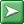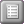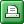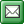﻿ Data Class Definition
Persistate

Data Class DefinitionData Class DefinitionData Class DefinitionA data class defines the set of values which an individual datum in that class can take.  A data class can be used to define the class of the members of an object class.  You can define a data class in a number of ways.

 ▪ A data class can simply define the primitive type of the data.  For example

An indicator takes boolean values.

 ▪ A data class can define a range of numerical values.  For example

A maximum lend count takes values from 1 to 6 .

 ▪ A data class can define the maximum length and optionally typical length of text or binary data.  For example

A title takes text values of length typically 25 maximum 100 .
A product code takes values of length 10 .

 ▪ A data class can define the precision and scale of decimal values.  For example

A temperature reading takes decimal values precision 12 scale 7;

 ▪ A data class can define a fixed set of values.  For example

A lend status takes values available, on loan, unavailable.

Syntax

DataClass = ( "a" | "an" ) DataClassName "takes" [ TypeName ] "values" [ ValueDefinition ] ;
DataClassName = <symbol> { <symbol> } ;
TypeName = <symbol> { <symbol> } ;
ValueDefinition = ( Bounds | Length | Precision | MultiValue ) ;
Bounds = "from" Number "to" Number ;
Number = ( <integer> | <floating> ) ;
Length = ( "to" | "of" ) "length" [ "typically" <integer> "maximum" ] <integer> ;
Precision = "precision" <integer> "scale" <integer> ;
MultiValue = Value { "," Value } ;
Value = <symbol> { <symbol> } ;

See Grammar Notation in Syntax Sections for an explanation of the format and symbols used here

Description

DataClass

This defines a data class.  The TypeName and the ValueDefinition are optional.  However, if you do not include a value definition you must include a type name, and vice versa.  If you include both, they must be compatible.

DataClassName

This is the name you give to the defined data class.  You use this name when you define an object class member with the data class.  If you define a MultiValue data class, the name must be a legal type name when converted to Pascal case, as this is used as the name of the generated struct.  The name must also be unique within the package.  No other data classes, object classes or view classes can have the same name.

TypeName

This defines the base type of the field which will hold a datum in the data class.  If you include a ValueDefinition, you don't normally need to include a TypeName, unless you want to specify a type which would not otherwise have been chosen automatically by default.  The following list gives the names of all the types you can use,

 ▪ boolean.  A single bit value
 ▪ byte,  An unsigned 8 bit integer.
 ▪ short integer.  A signed 16 bit integer
 ▪ integer.  A signed 32 bit integer.
 ▪ long integer  A signed 64 bit integer.
 ▪ float.  A 32 bit floating point number.
 ▪ double float.  A 64 bit floating point number.
 ▪ decimal.  In memory, a 128 bit fixed point number.  In persistent storage, a fixed point number with defined precision and scale.  If these are not supplied, defaults of 19 and 2 respectively are used.
 ▪ currency amount.  In memory a 128 bit fixed point number.  In persistent storage, the standard data type available for holding monetary values.
 ▪ datetime.  A value specifying a particular point in time.
 ▪ text.  In memory, a string.  In persistent storage, a standard fixed or variable length character field of the defined length.  If no length is supplied, the default of 1000 (variable) is used.
 ▪ long text.  In memory, a string.  In persistent storage, a field using the data type for extended length text.
 ▪ binary.  In memory, an unsigned byte array,  In persistent storage, a standard fixed or variable length binary field of the defined length. If no length is supplied, the default of 1000 (variable) is used.  In general you should always supply a length for binary fields.
 ▪ long binary.  In memory, an unsigned byte array.  In persistent storage, a field using the data type for extended length binary data.
 ▪ enumerated.  In memory, a struct generated by Persistate.  In persistent storage, a signed 32 bit integer.  This is the default type used when you define a MultiValue data class.
 ▪ combinable.  In memory, a struct generated by Persistate.  In persistent storage, a signed 32 bit integer.
 ▪ image.  In memory, a System.Drawing.Image.  In persistent storage, a field using the data type for extended length binary data.
 ▪ font.  In memory, a System.Drawing.Font.  In persistent storage,  a standard fixed or variable length character field of  length 100.  Persistate manages the conversion to and from the Font value.
 ▪ colour.  In memory, a System.Drawing.Color.  In persistent storage, a signed 64 bit integer.  Persistate manages the conversion to and from the Color value.

Bounds

This defines a range of numerical values.  If you do not define a TypeName, then the primitive type used by the data class will be set automatically to one of integer, long integer, float or double float, depending on the format and value of the supplied bounds.

Length

This defines the maximum length and optionally the typical length of text or binary data.  If you don't supply a TypeName, then the type will be set to Text.  The typical length, if set, is used by Persistate to determine the size of controls generated in a user interface to hold members of that class.

Precision

The precision and scale integers defined here are used to determine the total number of significant digits, and the number of digits after the decimal point respectively.  These values are used only when creating the fields used to hold the data in a persistent storage DBMS.  In memory, the System.Decimal type is used.

MultiValue

This defines a fixed set of values that object class members with this data class can take.  This type of data class definition is different to the others, in that for each such definition, Persistate generates a struct which implements the data class.  The struct contains a single 32 bit integer which holds the current value of the datum.  The struct contains constant definitions for each of the defined values, and also facilities to convert the struct to and from strings and integers.  There is a specialised TypeConverter which handles all such generated structs.

If you leave out the TypeName in a multi value definition, then the value stored in the struct is the ordinal position of the corresponding defined value, starting from 1.  The value 0 is left as "undefined".  You can also specify a TypeName of Combinable.  This works in a similar way to the Flags attribute for enumerations, and results in each value having its own bit position.